# modelCalibrationPlot

Scatter plot of predicted and observed EADs

Since R2023a

## Syntax

``modelCalibrationPlot(eadModel,data)``
``modelCalibrationPlot(___,Name=Value)``
``h = modelCalibrationPlot(ax,___,Name=Value)``

## Description

example

````modelCalibrationPlot(eadModel,data)` returns a scatter plot of observed vs. predicted exposure at default (EAD) data with a linear fit. `modelCalibrationPlot` supports comparison against a reference model. By default, `modelCalibrationPlot` plots in the EAD scale. ```

example

````modelCalibrationPlot(___,Name=Value)` specifies options using one or more name-value arguments in addition to the input arguments in the previous syntax. You can use the `ModelLevel` name-value argument to compute metrics using the underlying model's transformed scale. ```

example

````h = modelCalibrationPlot(ax,___,Name=Value)` specifies options using one or more name-value arguments in addition to the input arguments in the previous syntax and returns the figure handle `h`.```

## Examples

collapse all

This example shows how to use `fitEADModel` to create a `Tobit` model and then use `modelCalibrationPlot` to generate a scatter plot for predicted and observed EADs.

```load EADData.mat head(EADData)```
``` UtilizationRate Age Marriage Limit Drawn EAD _______________ ___ ___________ __________ __________ __________ 0.24359 25 not married 44776 10907 44740 0.96946 44 not married 2.1405e+05 2.0751e+05 40678 0 40 married 1.6581e+05 0 1.6567e+05 0.53242 38 not married 1.7375e+05 92506 1593.5 0.2583 30 not married 26258 6782.5 54.175 0.17039 54 married 1.7357e+05 29575 576.69 0.18586 27 not married 19590 3641 998.49 0.85372 42 not married 2.0712e+05 1.7682e+05 1.6454e+05 ```
```rng('default'); NumObs = height(EADData); c = cvpartition(NumObs,'HoldOut',0.4); TrainingInd = training(c); TestInd = test(c);```

Select Model Type

Select a model type for `Tobit` or `Regression`.

`ModelType =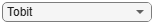"Tobit";`

Select Conversion Measure

Select a conversion measure for the EAD response values.

`ConversionMeasure =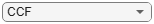"CCF";`

Create `Tobit` EAD Model

Use `fitEADModel` to create a `Tobit` model using the `TrainingInd` data.

```eadModel = fitEADModel(EADData(TrainingInd,:),ModelType,PredictorVars={'UtilizationRate','Age','Marriage'}, ... ConversionMeasure=ConversionMeasure,DrawnVar="Drawn",LimitVar="Limit",ResponseVar="EAD"); disp(eadModel);```
``` Tobit with properties: CensoringSide: "right" LeftLimit: NaN RightLimit: 1 ModelID: "Tobit" Description: "" UnderlyingModel: [1x1 risk.internal.credit.TobitModel] PredictorVars: ["UtilizationRate" "Age" "Marriage"] ResponseVar: "EAD" LimitVar: "Limit" DrawnVar: "Drawn" ConversionMeasure: "ccf" ```

Display the underlying model. The underlying model's response variable is the transformation of the EAD response data. Use the `'LimitVar'` and `'DrawnVar'` name-value arguments to modify the transformation.

`disp(eadModel.UnderlyingModel);`
```Tobit regression model, right-censored: EAD_ccf = min(Y*,1) Y* ~ 1 + UtilizationRate + Age + Marriage Estimated coefficients: Estimate SE tStat pValue __________ ________ _______ __________ (Intercept) 0.51514 0.11063 4.6565 3.3767e-06 UtilizationRate -1.5872 0.082441 -19.252 0 Age -0.0058079 0.00275 -2.112 0.034781 Marriage_not married 0.0077848 0.066893 0.11638 0.90736 (Sigma) 1.4689 0.020493 71.679 0 Number of observations: 2627 Number of left-censored observations: 0 Number of uncensored observations: 2626 Number of right-censored observations: 1 Log-likelihood: -6311.87 ```

EAD prediction operates on the underlying compact statistical model and then transforms the predicted values back to the EAD scale. You can specify the `predict` function with different options for the `'ModelLevel'` name-value argument.

```predictedEAD = predict(eadModel,EADData(TestInd,:),ModelLevel="ead"); predictedConversion = predict(eadModel,EADData(TestInd,:),ModelLevel="ConversionMeasure");```

For model validation, use `modelDiscrimination`, `modelDiscriminationPlot`, `modelCalibration`, and `modelCalibrationPlot`.

Use `modelDiscrimination` and then `modelDiscriminationPlot` to plot the ROC curve.

```ModelLevel =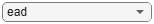"ead"; [DiscMeasure1,DiscData1] = modelDiscrimination(eadModel,EADData(TestInd,:),ModelLevel=ModelLevel); modelDiscriminationPlot(eadModel,EADData(TestInd, :),ModelLevel=ModelLevel,SegmentBy="Marriage");```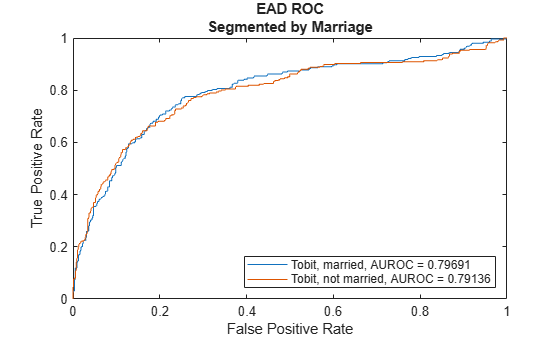Use `modelCalibration` and then `modelCalibrationPlot` to show a scatter plot of the predictions.

```YData =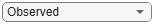"Observed"; [CalMeasure1,CalData1] = modelCalibration(eadModel,EADData(TestInd,:),ModelLevel=ModelLevel)```
```CalMeasure1=1×4 table RSquared RMSE Correlation SampleMeanError ________ _____ ___________ _______________ Tobit 0.33743 47299 0.58089 7285.1 ```
```CalData1=1751×3 table Observed Predicted_Tobit Residuals_Tobit __________ _______________ _______________ 44740 3337.3 41403 54.175 1654.8 -1600.6 987.39 11361 -10374 9606.4 9702.4 -95.942 83.809 2044.5 -1960.7 73538 70963 2575.3 96.949 846.22 -749.27 873.21 640.47 232.74 328.35 1357.3 -1028.9 55237 21710 33527 30359 29015 1344.2 39211 27048 12163 2.0885e+05 42571 1.6628e+05 1921.7 11162 -9240.8 15230 1542 13688 20063 6298.9 13764 ⋮ ```
`modelCalibrationPlot(eadModel,EADData(TestInd,:),ModelLevel=ModelLevel,YData=YData);`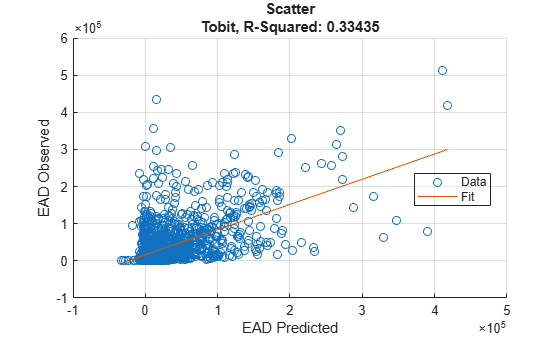This example shows how to use `fitEADModel` to create a `Beta` model and then use `modelCalibrationPlot` to generate a scatter plot for predicted and observed EADs.

```load EADData.mat head(EADData)```
``` UtilizationRate Age Marriage Limit Drawn EAD _______________ ___ ___________ __________ __________ __________ 0.24359 25 not married 44776 10907 44740 0.96946 44 not married 2.1405e+05 2.0751e+05 40678 0 40 married 1.6581e+05 0 1.6567e+05 0.53242 38 not married 1.7375e+05 92506 1593.5 0.2583 30 not married 26258 6782.5 54.175 0.17039 54 married 1.7357e+05 29575 576.69 0.18586 27 not married 19590 3641 998.49 0.85372 42 not married 2.0712e+05 1.7682e+05 1.6454e+05 ```
```rng('default'); NumObs = height(EADData); c = cvpartition(NumObs,'HoldOut',0.4); TrainingInd = training(c); TestInd = test(c);```

Select Model Type

Select a model type for `Beta`.

`ModelType =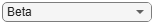"Beta";`

Select Conversion Measure

Select a conversion measure for the EAD response values.

`ConversionMeasure =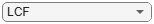"LCF";`

Create `Beta` EAD Model

Use `fitEADModel` to create a `Beta` model using the `TrainingInd` data.

```eadModel = fitEADModel(EADData(TrainingInd,:),ModelType,PredictorVars={'UtilizationRate','Age','Marriage'}, ... ConversionMeasure=ConversionMeasure,DrawnVar="Drawn",LimitVar="Limit",ResponseVar="EAD"); disp(eadModel);```
``` Beta with properties: BoundaryTolerance: 1.0000e-07 ModelID: "Beta" Description: "" UnderlyingModel: [1x1 risk.internal.credit.BetaModel] PredictorVars: ["UtilizationRate" "Age" "Marriage"] ResponseVar: "EAD" LimitVar: "Limit" DrawnVar: "Drawn" ConversionMeasure: "lcf" ```

Display the underlying model. The underlying model's response variable is the transformation of the EAD response data. Use the `'LimitVar'` and `'DrawnVar'` name-value arguments to modify the transformation.

`disp(eadModel.UnderlyingModel);`
```Beta regression model: logit(EAD_lcf) ~ 1_mu + UtilizationRate_mu + Age_mu + Marriage_mu log(EAD_lcf) ~ 1_phi + UtilizationRate_phi + Age_phi + Marriage_phi Estimated coefficients: Estimate SE tStat pValue __________ _________ ________ __________ (Intercept)_mu -0.65566 0.11484 -5.7093 1.2616e-08 UtilizationRate_mu 1.7014 0.078094 21.787 0 Age_mu -0.0055901 0.0027603 -2.0252 0.042949 Marriage_not married_mu -0.012577 0.052098 -0.24141 0.80926 (Intercept)_phi -0.50131 0.094625 -5.2979 1.2686e-07 UtilizationRate_phi 0.39731 0.066707 5.956 2.9303e-09 Age_phi -0.001167 0.0023161 -0.50387 0.6144 Marriage_not married_phi -0.013275 0.042627 -0.31143 0.7555 Number of observations: 2627 Log-likelihood: -3140.21 ```

EAD prediction operates on the underlying compact statistical model and then transforms the predicted values back to the EAD scale. You can specify the `predict` function with different options for the `'ModelLevel'` name-value argument.

```predictedEAD = predict(eadModel,EADData(TestInd,:),ModelLevel="ead"); predictedConversion = predict(eadModel,EADData(TestInd,:),ModelLevel="ConversionMeasure");```

For model validation, use `modelDiscrimination`, `modelDiscriminationPlot`, `modelCalibration`, and `modelCalibrationPlot`.

Use `modelDiscrimination` and then `modelDiscriminationPlot` to plot the ROC curve.

```ModelLevel ="ead"; [DiscMeasure1,DiscData1] = modelDiscrimination(eadModel,EADData(TestInd,:),ModelLevel=ModelLevel); modelDiscriminationPlot(eadModel,EADData(TestInd, :),ModelLevel=ModelLevel,SegmentBy="Marriage");```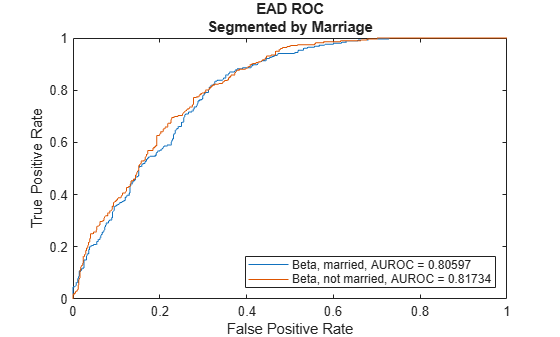Use `modelCalibration` and then `modelCalibrationPlot` to show a scatter plot of the predictions.

```YData ="Observed"; [CalMeasure1,CalData1] = modelCalibration(eadModel,EADData(TestInd,:),ModelLevel=ModelLevel)```
```CalMeasure1=1×4 table RSquared RMSE Correlation SampleMeanError ________ _____ ___________ _______________ Beta 0.38655 43817 0.62173 -7393.4 ```
```CalData1=1751×3 table Observed Predicted_Beta Residuals_Beta __________ ______________ ______________ 44740 18039 26701 54.175 10560 -10506 987.39 15551 -14564 9606.4 8407.7 1198.8 83.809 33318 -33234 73538 52120 21418 96.949 6598.1 -6501.2 873.21 5471.1 -4597.9 328.35 7335 -7006.6 55237 32580 22658 30359 21563 8796.4 39211 33177 6033.6 2.0885e+05 1.2586e+05 82987 1921.7 23319 -21397 15230 6565.9 8664 20063 11075 8987.5 ⋮ ```
`modelCalibrationPlot(eadModel,EADData(TestInd,:),ModelLevel=ModelLevel,YData=YData);`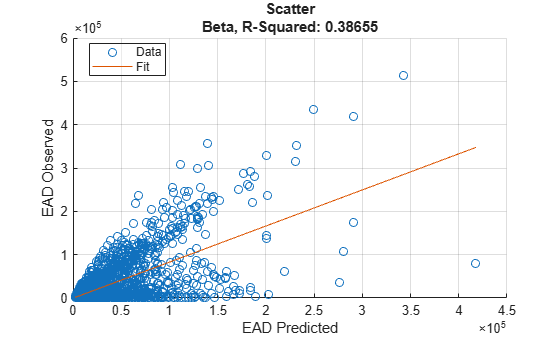## Input Arguments

collapse all

Exposure at default model, specified as a previously created `Regression`, `Tobit`, or `Beta` object using `fitEADModel`.

Data Types: `object`

Data, specified as a `NumRows`-by-`NumCols` table with predictor and response values. The variable names and data types must be consistent with the underlying model.

Data Types: `table`

(Optional) Valid axis object, specified as an `ax` object that is created using `axes`. The plot will be created in the axes specified by the optional `ax` argument instead of in the current axes (gca). The optional argument `ax` must precede any of the input argument combinations.

Data Types: `object`

### Name-Value Arguments

Specify optional pairs of arguments as `Name1=Value1,...,NameN=ValueN`, where `Name` is the argument name and `Value` is the corresponding value. Name-value arguments must appear after other arguments, but the order of the pairs does not matter.

Example: `modelCalibrationPlot(eadModel,data(TestInd,:),DataID=Testing,XData='residuals',YData='residuals')`

Data set identifier, specified `DataID` and a character vector or string. The `DataID` is included in the output for reporting purposes.

Data Types: `char` | `string`

Model level, specified as `ModelLevel` and a character vector or string.

Note

`Regression` models support all three model levels, but a `Tobit` or `Beta` model supports model levels only for `"ead"` and `"conversionMeasure"`.

Data Types: `char` | `string`

EAD values predicted for `data` by the reference model, specified as `ReferenceEAD` and a `NumRows`-by-`1` numeric vector. The scatter plot output is plotted for both the `eadModel` object and the reference model.

Data Types: `double`

Identifier for the reference model, specified as `ReferenceID` and a character vector or string. `ReferenceID` is used in the scatter plot output for reporting purposes.

Data Types: `char` | `string`

Data to plot on x-axis, specified as `XData` and a character vector or string for one of the following:

• `'predicted'` — Plot the predicted EAD values in the x-axis.

• `'observed'` — Plot the observed EAD values in the x-axis.

• `'residuals'` — Plot the residuals in the x-axis.

• VariableName — Use the name of the variable in the `data` input, not necessarily a model variable, to plot in the x-axis.

Data Types: `char` | `string`

Data to plot on y-axis, specified as `YData` and a character vector or string for one of the following:

• `'predicted'` — Plot the predicted EAD values in the y-axis.

• `'observed'` — Plot the observed EAD values in the y-axis.

• `'residuals'` — Plot the residuals in the y-axis.

Data Types: `char` | `string`

## Output Arguments

collapse all

Figure handle for the scatter and line objects, returned as handle object.

collapse all

### Model Calibration Plot

The `modelCalibrationPlot` function returns a scatter plot of observed vs. predicted loss given default (EAD) data with a linear fit and reports the R-square of the linear fit.

The `XData` name-value pair argument allows you to change the x values on the plot. By default, predicted EAD values are plotted in the x-axis, but predicted EAD values, residuals, or any variable in the `data` input, not necessarily a model variable, can be used as x values. If the selected `XData` is a categorical variable, a swarm chart is used. For more information, see `swarmchart`.

The `YData` name-value pair argument allows users to change the y values on the plot. By default, observed EAD values are plotted in the y-axis, but predicted EAD values or residuals can also be used as y values. `YData` does not support table variables.

The linear fit and reported R-squared value always correspond to the linear regression model with the plotted y values as response and the plotted x values as the only predictor.

 Baesens, Bart, Daniel Roesch, and Harald Scheule. Credit Risk Analytics: Measurement Techniques, Applications, and Examples in SAS. Wiley, 2016.

 Bellini, Tiziano. IFRS 9 and CECL Credit Risk Modelling and Validation: A Practical Guide with Examples Worked in R and SAS. San Diego, CA: Elsevier, 2019.

 Brown, Iain. Developing Credit Risk Models Using SAS Enterprise Miner and SAS/STAT: Theory and Applications. SAS Institute, 2014.

 Roesch, Daniel and Harald Scheule. Deep Credit Risk. Independently published, 2020.